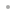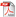MathCode C++MathCode C++FeaturesExamplesQ&ANew in Version 1.4Download ProductManualDownload Product Information SheetBuy OnlineRequest a Free Trial# Examples

Simplest Example
This example shows the core functionality of MathCode C++ by compiling a trivial Mathematica function that adds two numbers. It shows how the compilation is performed and how the compiled code can be called directly from within Mathematica.

Code Generation of a Symbolic Matrix
This notebook demonstrates how MathCode C++ can be used to generate C++ code to efficiently compute a large matrix with symbolic entries. The matrix in this case is a Hessian of a given function, which is an important entity to compute efficiently. Hessians have many applications, e.g., in optimization algorithms.

The Seesaw/Pendulum Process
This notebook illustrates how to build a dynamic model of a physical system and then generate efficient code for simulation.

Find Minimum
The function FindMinimum[] finds a local minimum of a given function and a given starting value. A function cannot be translated by MathCode directly when the argument is a symbolic expression. Also, numerical algorithms for minimization are not included in the MathCode runtime library. The solution is to write a Mathematica function that replicates the FindMinimum[] operation using the compilable subset. This notebook illustrates how to do that for a function with one argument.